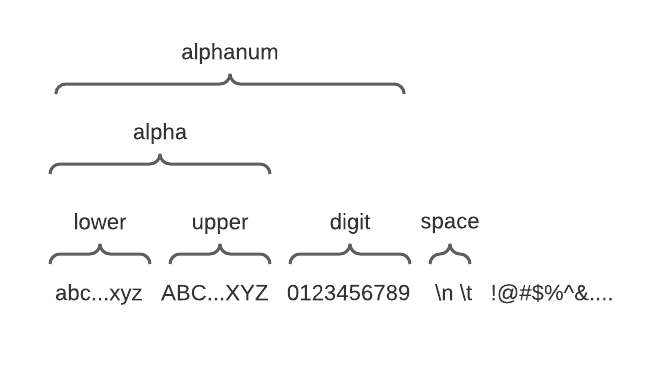Computer Science

# Strings

We have previously seen strings. Here we will show you quite a few more details.

A string is surrounded by single or double quotes:

``````name = 'Mariano'
food = "homemade lasagna is the best"``````

You can get the length of a string with `len()`, the same as the length of a list:

``````if __name__ == '__main__':
name = 'Mariano'
food = "homemade lasagna is the best"
print(len(name))
print(len(food))
print(f"'{name}' has {len(name)} characters and '{food}' has {len(food)} characters.")``````

This will print:

``````7
28
'Mariano' has 7 characters and 'homemade lasagna is the best' has 28 characters.``````

## Concatenating strings

You can use several operations with strings. If you add strings, they are concatenated. So this:

``result = 'fire' + 'place'``

will set `result` to `'fireplace'`.

Often you will need to take an existing string and add on to it:

``````result = 'hello'
result = result + ' '
result = result + 'there'``````

Now `result` references `"hello there"`.

You can do this more easily with `+=`:

``````result = 'hello'
result += ' '
result += 'there'``````

## Accumulating a result with concatenation

Concatenation is particularly useful when you want to accumulate a result. For example:

``````def collect_words():
result = ''
while True:
word = input('Enter a word: ')
if word == '':
break
if result != '':
result += ' '
result += word

return result

if __name__ == '__main__':
print(collect_words())``````

In this code we use the `result` variable to accumulate all of the words a person types. We use the `+=` to add in each word. We also use `+=` to add a space between the words. We use one litle trick to do this. Notice:

``````if result != '':
result += ' '``````

We don’t add the space if `result` is an empty string. This prevents us from adding a space at the start of `result` before we have concatenated any words. This prevents us from adding space the first time through the loop.

When you run this code, you might see:

``````Enter a word: Yer
Enter a word: a
Enter a word: wizard
Enter a word: Harry
Enter a word:
Yer a wizard Harry``````

## Iterating over the characters in a string

You will recall that we have iterated over lists of numbers:

``````numbers = [1, 2, 3]
for number in numbers:
print(number)``````

lists of strings:

``````names = ["Mario", "Maria", "Magda"]
for name in names:
print(name)``````

and even lists of tuples:

``````players = [('John', 42), ('Emma', 33), ('Jose', 10), ('Rosaria', 18)]
for name, jersey in players:
print(f"{name} wears jersey number {jersey}")``````

We can likewise iterate over the characters in a string:

``````line = 'what a life'
for character in line:
print(character)``````

This will print out each character, including spaces, on a separate line:

``````w
h
a
t

a

l
i
f
e``````

## Functions for testing strings

Following are some of the functions you can use to test strings:

• `islower()`: returns true if all characters are lowercase
• `isupper()`: returns true if all characters are uppercase
• `isalpha()`: true if all characters are alphabetic
• `isdigit()`: true if all characters are digits
• `isalnum()`: true if all characters are alphanumberic (alphabetic or digits)
• `isspace()`: true if all characters are white space (space, tab, newline)For example:

``````'abc'.islower()    # True
'abC'.islower()    # False
'ABC'.isupper()    # True
'ABc'.isupper()    # False
'a'.isalpha()     # True
'ab3'.isalpha()   # False
'8'.isdigit()     # True
'89a'.isdigit()   # False
'89a'.isalnum()   # True
' \t\n'.isspace() # True``````

All of these functions work on variables that reference strings, whether those strings are one or many characters long. For example:

``````password = 'adam123'
print('Yes')
else:
print('No')``````

This will print `Yes`.

## Capitalization

These functions will change capitalization:

• `upper()`: returns a new string that is all uppercase
• `lower`: returns a new string that is all lowercase

For example:

``````
'abc'.upper()      # 'ABC'
'ABC'.lower()      # 'abc'``````

## No spaces

Write a function that replaces all space characters in a string with dashes.

Work with a friend to write this code. This should take only one function. You can find starter code in `no_spaces.py`.Here is a solution:

``````def no_spaces(text):
result = ''
for c in text:
if c.isspace():
c = '-'
result += c
return result``````

We use the accumulate pattern to build up the result string in the variable called `result`. As we loop through the string, charcter by character, we change the character to be a `'-'` if it is a space. We then append the character to the result string.

## Replacing numbers

Write a function that replaces every number in a string with a `?`.

Work with a friend to write this code. This should take only one function. You can find starter code in `replace_numbers.py`.Here is a solution:

``````def no_numbers(text):
result = ''
for char in text:
if char.isdigit():
result += '?'
else:
result += char
return result``````

We again use the accumulate pattern. Here instead of changing the character to `?` if it is a number, we append a `?`. Notice that this means we need to use `else` and have two places where we accumulate into the `result` string.

This problem is very similar to the `No spaces`, and either solution could be written in either style.

## Sum digits

Write a function that sums all the digits in a string.

Work with a friend to write this code. This should take only one function. You can find starter code in `sum_digits.py`.Here is a solution:

``````def sum_digits(text):
"""Add all the digits found in the `text`."""
total = 0
for c in text:
if c.isdigit():
total += int(c)
This also uses the accumulate pattern. Surprise! :-) We use `total` to keep track of the total. And we have to use `int()` to convert the character to an integer that we can add into `total`.